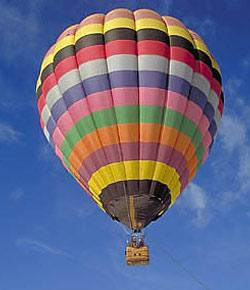# Gas Laws Quiz #1

8 Questions | Total Attempts: 706SettingsQuiz on gas laws # 1 includes questions on Boyle's Law. Charles' Law, Lussac's Law, and the Combined Gas Law. Identify the law you need to solve the problem and solve the problem. Use classroom rules for rounding and include units in your answer. Example: If 6 liters of a gas at 2. 0 atm is compress the gas to have a new volume of 5 liters, what is the new pressure? This is a Boyle's Law Problem so P1V1 = P2V2(2. 0)(6) = (P2)(5)P2 = 2. 4Express the answer as 2. 4 atmNote rounding is important numbers greater than 1 are rounded to the tenths place (1 = 1. 0, 5. 85 = 5. 9 etc. . . ) and numbers less than 1 are rounded to one place after the first non zero (0. 000567 = 0. 00057, 02875 = 0. 29)Units are expressed after the number as above. Include a

• 1.
A gas in a balloon that has a volume of  5 L is at a pressure of 0.7atm. The gas is able to expand at a constant temp of 10 degrees Celsius until the pressure is at 0.5atm. What is the final volume of the box?
• 2.
A 3.5 L sample of an unknown gas is at a pressure of 900kPa. If the pressure increases to 1013 kPa at a constant temperature of 150 degrees kelvin, What will the new volume be?
• 3.
A gas occupies 15 L at a pressure of 80 mm-Hg. What is the volume when the pressure is increased to 525 mm-Hg?
• 4.
A gas occupies 10 L at an unknown pressure. What is the initial pressure, if the final volume is 5L and the pressure is 5atm?
• 5.
. A gas occupies 15 L at a pressure of 5 atm. What is the volume when the pressure is increased to 10 atm but the temperature remains at 273 degrees kelvin?
• 6.
A gaseous sample of carbon dioxide occupies a volume of 3.27 L at a temperature of 20 degrees Celsius. If the pressure remains constant, what is the temperature in Kelvin if the volume lowers to 3L?
• 7.
What is the temperature in Kelvin when a gas that occupies 1L at 15 degrees Celsius is compressed to .5L?
• 8.
A 700 ml sample of helium is warmed from 60 to 80 degrees Celsius. What is the new volume in mL of the sample if the pressure remains as a constant, of 5 atm.
Related TopicsBack to top